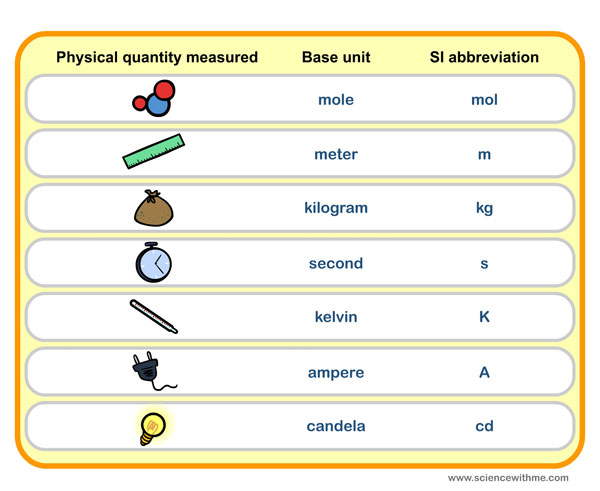S.I. stands for Systeme Internationale, which is also known as the International System of Units.

You may not notice this but we always use this as a form of measurement. SI units have been accepted around the world as an ideal replacement to other metric systems that may cause confusion and uncertainty among people. Today, people from all countries follow this metric system, and that includes me and you!

### How did S.I. Units come about?

A long time ago, people from different countries had different ways of measuring things, which made it harder for them to trade. People need to measure what they buy and exchange for them to prosper, but not having a common and correct way of measuring was a problem. Finally, scientists came up with a unit of measurement that can replace all other existing ways we measure objects. This is how the SI unit of measurement came about. Using the SI unit of measurement helped everyone understand and communicate well doing business. People realized how practical it was to use only one measurement system for every kind of unit. They can now use one unit to measure the weight of a sack of potatoes and a slab of meat, and made it easier to compare their value. It became so useful that everybody started to use SI units because it allowed them to compute in an accurate and simple manner.

### What do we measure in S.I. Units?

Scientists decided to set this system of measurement to measure nearly everything that’s valuable and important. From the seven basic units of S.I., we can now compute for mass, length, time, temperature, amount or quantity of a substance in matter, brightness of an object or luminous intensity and the flow of electric currents. Even if we use other forms of measurement, we can easily convert these into SI units by following the conversion table.

### How do we measure in S.I. Units?

S.I. units are so precise that these are used in all scientific measurements. There are seven basic units, and then we add prefixes for sub-units. Each sub-unit originates from the base unit, so it’s easier to identify the type of measurement used (for example, adding the prefix centi to the basic unit meter = centimeter, which is one hundredth of a meter). Listed below are the seven SI basic units of measurement.• Meter (abbreviated as m). The meter is used to calculate distance or length. When scientists first thought of the meter, it was described as one ten-millionth of the distance between the equator and the North Pole. Comparing with other metric systems, 1 yard is exactly 0.9144 of a meter.
• Kilogram (abbreviated as kg). The kilogram is used to compute for mass, which is the measurement of the quantity of matter in any given object. In science the kilogram is used to measure mass, but for us we use it to measure both mass and weight. One kilogram is actually a thousand grams, and although gram can be considered the chief unit, the kilogram (prefix kilo + gram) is used to define measurement for objects with considerable size. In real life, we use the kilogram more to shorten descriptions of measurement that may be too long for comfort (example, 22,000 grams = 22 kilograms).
• Second (abbreviated as s). The second is the basic unit of measurement for time. Because we now have a way to observe time in its smallest unit or form, this was helpful especially in sports and science. Before, 1 second was first used to describe the time spend in the earth’s rotation. Today 1 second is 1/60 of a minute based on the numerical conversion.
• Kelvin (abbreviated as K). The kelvin is used to compute the amount of heat in a certain object. We may be not very familiar with using the kelvin to measure temperature, but it is called the absolute scale because the temperature is only stated in positives (no Kelvin measurement uses the negative sign).
• Mole (abbreviated as mol). To compute for the quantity of substance within an object, SI uses the mole as its basic unit of measurement. Because there are no other available units used to measure the amount of substance within matter, we may also not be aware of using the mole as a unit of measurement, but this is the general term used by scientists.
• Candela (abbreviated as cd). The candela is used to measure the brightness or luminous intensity of an object. You can use this to compute for how much light an object emits, or the amount it glows, or simply how bright it is.
• Ampere (abbreviated as A). Amperes or more commonly called amps, is used to measure the electric current. Amps can be used to compute for how much electricity runs in a given object, so all conductors of electricity uses amps to calculate the flow of the current.

### Why is it important to use S.I. Units?

Because using SI units allow us to measure objects or matter in a more simple, straightforward and yet accurate manner, calculating is effortless for everyone. Using an exact way to measure things is way better, and of course easier and more reliable than using your arms or legs to explain the distance between two points. Isn’t it fun to learn about SI units? Now you can easily compare one object with another by using the SI unit for mass (kilogram), or know how far it is to go to a certain place just by using the SI unit for length or distance (meter). Want to learn more about the SI Units? Check out the free previews from The King Activity Set! You can also download our free worksheet and coloring page all about SI Units!